# Grade - math word problems

#### Number of examples found: 5310

• Right triangleCalculate the length of the remaining two sides and the angles in the rectangular triangle ABC if a = 10 cm, angle alpha = 18°40'.
• PerformanceTwo masons with the same performance would have made of plaster for 6 days. One of them, however, has increased its daily performance by 50%. How long would take they now to make plaster together?
• Tangent 3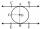In a circle with centre O radius is 4√5 cm. EC is the tangent to the circle at point D. Segment AB IS THE DIAMETER of given circle. POINT A is joined with POINT E and POINT B is joined with POINT C. Find DC if BC IS 8cm.
• Three granaries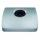Three granaries hold 7,800 metric cent (q) of grain. In the first, 70 tonnes of grain was more than the second and in the third it was 120 tonnes less than in the second. How many grain were in each granary?
• Cost reductionTwo MP3 players whose price was equal to originally have been discounted the first by 20%, the second by 35%. After the price reduction was the difference in their prices 750, - CZK. What was the original price of each of the two players?
• Average speed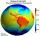What is the average speed you have to move the way around the world in 80 days? (Path along the equator, round to km/h).
• Working time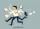At 10 hours work shift, work is done in 16 days. How many days will the work be done at 8 hours shifts?
• Prism - boxThe base of prism is a rectangle with a side of 7.5 cm and 12.5 cm diagonal. The volume of the prism is V = 0.9 dm3. Calculate the surface of the prism.
• Alcohol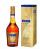How many cubic centimeters of 96% alcohol is needed to obtain 0.75 liter of 40% alcohol?
• Salary in enterprise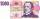The average salary in the company is 27 000 CZK, 30% of workers have the lowest average income of 19 thousand CZK. There were an increase in the salary in this group by 2%. How much % increased the average salary across the company?
• Work at plant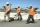Handyman has vowed to do repair work at the plant for 25 days. However, work had to be shortened and therefore gained helper. Together they made all the corrections for 13 1/3 days. How long the work would take helper himself?
• Triangle ABCTriangle ABC has side lengths m-1, m-2, m-3. What has to be m to be triangle a) rectangular b) acute-angled?
• Broken treeThe tree was 35 meters high. The tree broke at a height of 10 m above the ground. Top but does not fall off it refuted on the ground. How far from the base of the tree lay its peak?
• Jane and Miro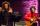Jane brother Miro is 42 years. And he is three times old as it was Jane when Miro was for so many years as there are now Jane. How old is Jane?
• Mother and daughter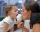Three years ago mother was three times older than daughter. After nine years she will be only twice old. How old is mother (and daughter)?
• Snowman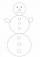In a circle with a diameter 50 cm are drawn 3 circles /as a snowman/ where: its diameters are integers, each larger circle diameter is 3 cm larger than the diameter of the previous circle. Determine snowman height if we wish highest snowman.
• Lie/do not lie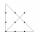The function is given by the rule f(x) = 8x+16. Determine whether point D[-1; 8] lies on this function. Solve graphically or numerically and give reasons for the your answer.
• Salary raiseThe monthly salary was 620 Eur. During the year it was raised to 727 Eur. Calculate the month from salary was increased that employee earned 7547 Eur during the whole year.
• Nádoba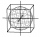Nádoba tvaru kostky je naplněna vodou do poloviny své výšky. Pokud dolijeme 20 l vody, bude nádoba naplněna do tří čtvrtin své výšky. Jaký je objem celé nádoby?
• Bonuses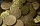Five employees of the company were paid bonuses so that each successor received a 550 USD less than the previous employee. How much did everyone get, if a total of USD 11,000 has paid?

Do you have an interesting mathematical word problem that you can't solve it? Submit math problem, and we can try to solve it.

We will send a solution to your e-mail address. Solved examples are also published here. Please enter the e-mail correctly and check whether you don't have a full mailbox.

Please do not submit problems from current active competitions such as Mathematical Olympiad, correspondence seminars etc...